# Getting Started with Image Processing using MATLAB

Published  November 14, 2018   3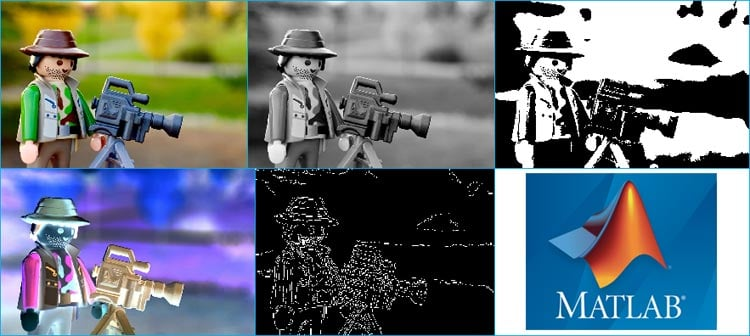Imagine pointing your camera to some object and the camera tells you the name of that object, yes, Google Lens in Android smart phones is doing the same thing using Image Processing. This gives computer a vision to detect and recognize the things and take actions accordingly. Image processing has lot of applications like Face detection & recognition, thumb impression, augmented reality, OCR, Barcode scan and many more. There are lot of softwares available for image processing, among them MATLAB is the most suitable to start with.

MATLAB can perform many advance image processing operations, but for Getting started with Image processing in MATLAB, here we will explain some basic operations like RGB to Gray, rotate the image, binary conversion etc. You can further make automated programs for noise removal, image clarity, filtering by using the functions explained in this tutorial.

Before proceeding further, if you are new to MATLAB you can check our previous MATLAB tutorials for better understating:

In MATLAB, as always, there are two ways to perform any image processing algorithm, one is by directly entering the command in the editor/command window and other is by creating a GUI for the same. Here, we will show you both the methods to perform basic operations of image processing in MATLAB.

### 1. Image Processing by using MATLAB Editor Window

Now, we will write the code for performing some basic operations of image processing in the editor window. To get familiar with all the basic terminology used in MATLAB follow the link. Copy and paste the below code in the editor window,

```a = imread('F:\circuit digest\image processing using matlab\camerman.jpg');
subplot(2,3,1);
imshow(a);

b = rgb2gray(a);
subplot(2,3,2);
imshow(b);

c = im2bw(a);
subplot(2,3,3);
imshow(c);

subplot(2,3,4);
imshow(d);

e = a;
e=rgb2gray(e);
subplot(2,3,5);
imhist(e);

imfinfo('F:\circuit digest\image processing using matlab\beard-man.jpg')

[height, width, colour_planes] = size(a)

%colormap('spring')```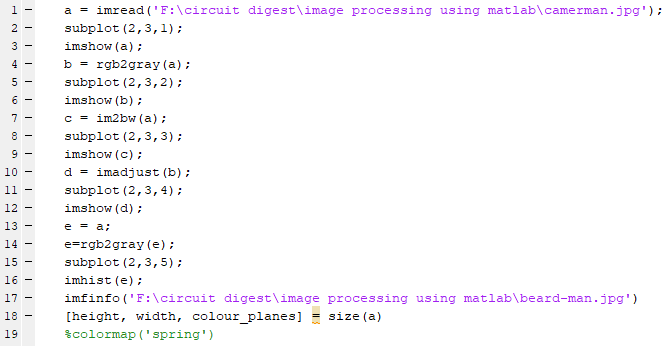In variable ‘a’, we are importing image using command imread(‘filename’) and then making a plot of ‘2’ row and ‘3’  column using subplot(row, column, position) and displaying the imported image on position ‘1’. To show the image we use command imshow(‘filename’).

Below are few commands to perform some basic processing on uploaded image:

• In variable ‘b’, we are converting the RGB image into grayscale intensity image by using the command rgb2gray(‘filename’) and displaying it in plot on position ‘2’.
• In variable ‘c’, we are converting the image into binary image or you can say in format of ‘0’ (black) and ‘1’ (white) by using the command im2bw(‘filename’) and displaying it in plot on position ‘3’.
• In variable ‘d’, we are adjusting or mapping the grayscale image intensity values by using the command imadjust(‘filename’) and displaying it in plot on position ‘4’.
• In variable ‘e’, we are plotting the histogram of the grayscale image by using the command imhist(‘filename’) and displaying it in plot on position ‘5’. For plotting the histogram you always have to convert the image into grayscale and then you will be able to see the histogram of that graphic file.
• Imfinfo(‘filename with location’) command is used to display information about the graphical file.
• [height, width, colour_planes] = size(‘filename’) command is used to display the size and color planes of a particular graphic file.
• colormap('spring') is used to change the type of colormap of graphic file. Here, in my code I set this command as comment but you can use it by removing the percentage sign. There are many types of color in MATLAB like Jet, HSV, Hot, Cool, Summer, Autumn, Winter, Gray, Bone, Copper, Pink, Lines and spring.

Like these, there are number of commands in MATLAB which can be used to perform different tasks, you can check the image processing functions in MATLAB by following the link.

### Creating MATLAB Graphical User Interface for Image Processing

For building a GUI (Graphical User Interface) for Image Processing launch the GUI by typing the below command in the command window.

`guide`

A popup window will open, then select new blank GUI as shown in below image,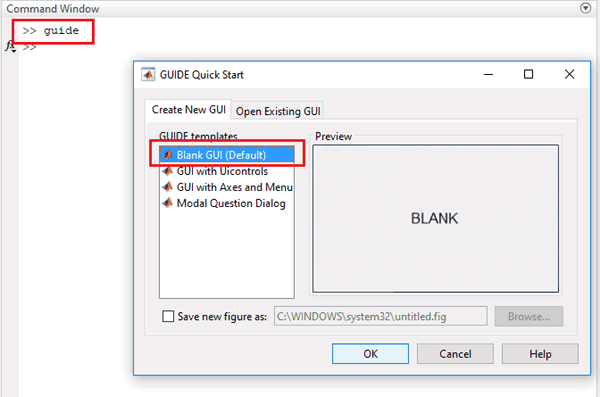Now we have to choose number of pushbuttons (every pushbutton will perform different task) and one axis to display image.

To resize or to change the shape of the Pushbutton or Axes, just click on it and you will be able to drag the corners of the button. By double-clicking on any of these you will be able to change the color, string, tag and other options of that particular button. After customization it will look like this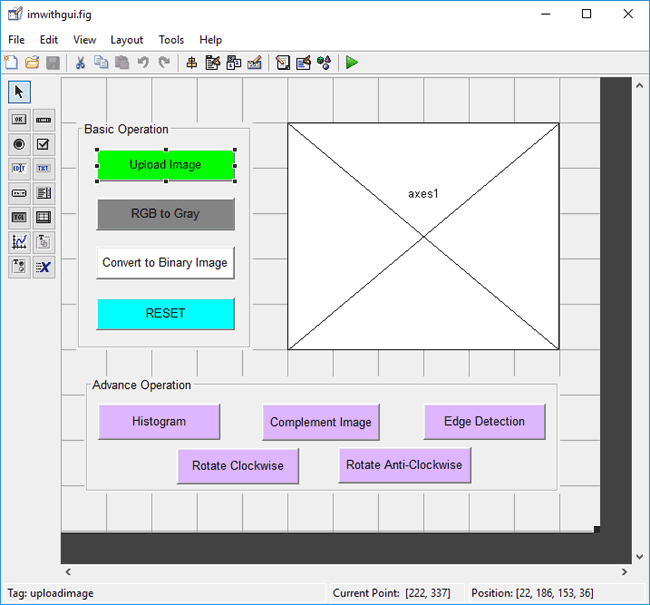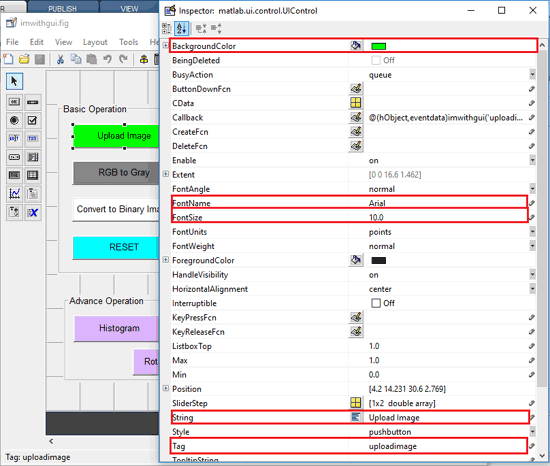You can customize the buttons as per your choice. Now when you save this, a code is generated in the Editor window of MATLAB. Edit the generated code to set the task for different pushbuttons. Below we have edited the MATLAB code.

### MATLAB GUI Code for Image Processing

Complete MATLAB code for Image Processing using MATLAB GUI, is given at the end of this project. Further we are including the GUI file (.fig) and code file(.m) here for download, using which you can customize the buttons or Axes size as per your requirement. We have edited the generated code as explained below.

In the ‘uploadimage’ function, copy and paste the below code to insert the file from the PC. Here, command uigetfile(‘image extension type’) is used for importing image in the MATLAB GUI. Read that file using command imread() and then display it with command imshow() on axes1 using axes(handles.axes1). Now, with the command setappdata(), store the variable in the GUI so the variable will be accessible to one part of the GUI to the other part of the GUI.

```a=uigetfile('.jpg')
axes(handles.axes1);
imshow(a);
setappdata(0,'a',a)```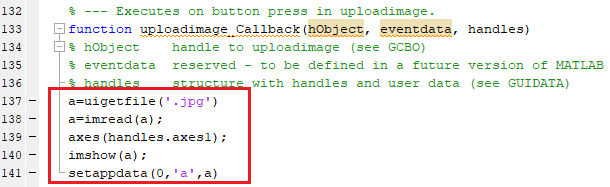Now, in every function you will see command getappdata() which is used to retrieve data which is stored using the setappdata() in the GUI.

Here we will explain eight commonly used functions in image processing

 S. No. Command Button Name Task to be Performed 1. uigetfile() Upload Image Click to import image from Disk 2. rgb2gray() RGB to Gray Click to convert RGB image into grayscale 3. im2bw() Convert to Binary Image Click to convert the image into binary 4. - RESET Click to reset the image as original 5. imhist() Histogram Click to see the histogram of the image 6. imcomplement() Complement Image Click to check the complement image 7. edge(filename,method) Edge Detection Click to detect the edges in the image 8. imrotate(filename,angle) Rotate Clockwise Click to rotate the image into the clockwise direction 9. imrotate(filename,angle) Rotate Anti-Clockwise Click to rotate the image into the anti-clockwise direction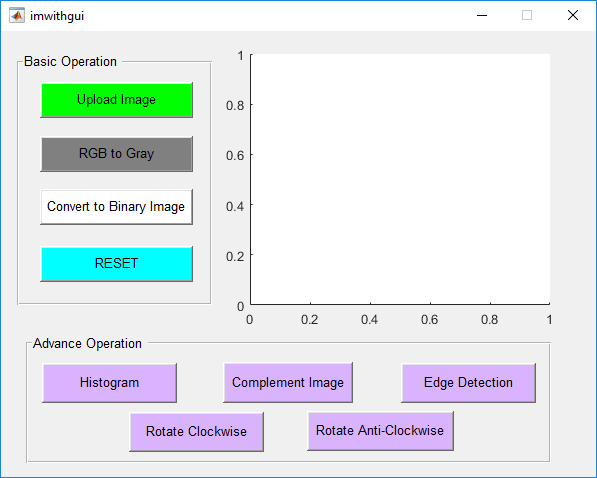1.      Convert RGB image into grayscale

In the ‘rgb2gray’ function, copy and paste the below code to convert the RGB image into grayscale by using command rgb2gray().

```a=getappdata(0,'a');
agray=rgb2gray(a);
axes(handles.axes1);
imshow(agray);```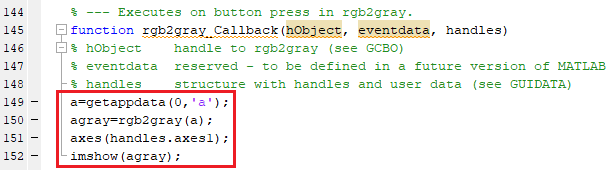2.      Convert to Binary Image

In the ‘im2bw’ function, copy and paste the below code to convert the image into binary image or you can say in format of ‘0’ (black) and ‘1’ (white) by using command im2bw().

```a=getappdata(0,'a');
abw=im2bw(a);
axes(handles.axes1);
imshow(abw);```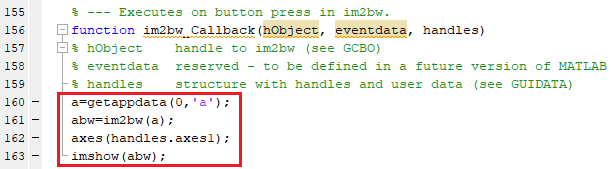3.      Reset to Original Image

In the ‘reset’ function, copy and paste the below code to reset the edited image into the original image.

```a=getappdata(0,'a');
axes(handles.axes1);
imshow(a);```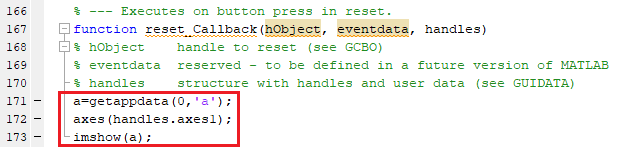4.      Plot Image Histogram

In the ‘histogram’ function, copy and paste the below code to plot the histogram of the grayscale image by using the command imhist(‘filename’) and display it in on axes1. For plotting the histogram you always have to convert the image into grayscale and then you will be able to see the histogram of that graphic file.

```a=getappdata(0,'a');
ahist=a;
ahist=rgb2gray(ahist);
axes(handles.axes1);
imhist(ahist);```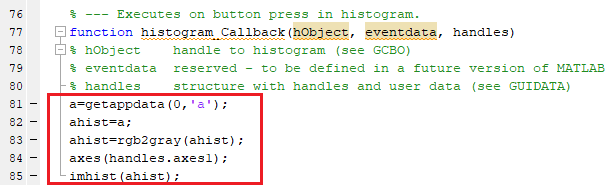5.      Convert to Complement Image

In the ‘complementimage’ function, copy and paste the below code to see the complement of the inserted graphic file by using command imcomplement().

```a=getappdata(0,'a');
acomp=a;
acomp=imcomplement(acomp);
axes(handles.axes1);
imshow(acomp);```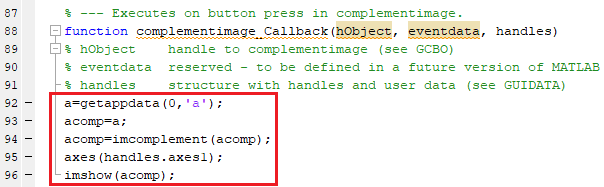6.      Edge Detection using Canny Method

In the ‘edge’ function, copy and paste the below code to detect and find edges in grayscale image by using command edge(‘filename’,’method’). In the place of method you can choose among these three, Canny, Prewitt and montage. We are using Canny method for edge detection. Also you cannot detect the edge directly from the original image, first you have to convert it into grayscale and then you can able to detect the edges.

```a=getappdata(0,'a');
aedge=a;
aedge=rgb2gray(aedge);
aedge=edge(aedge,'Canny')'
axes(handles.axes1);
imshow(aedge);
```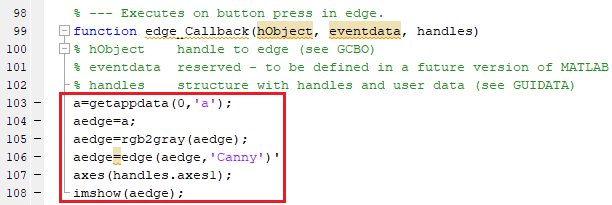7.      Rotate Image Clockwise

In the ‘clockwise’ function, copy and paste the below code to rotate the image in the clockwise direction by using command imrotate(filename,‘angle’)

```a=getappdata(0,'a');
aclock=a;
aclock=imrotate(aclock,270);
axes(handles.axes1);
imshow(aclock);```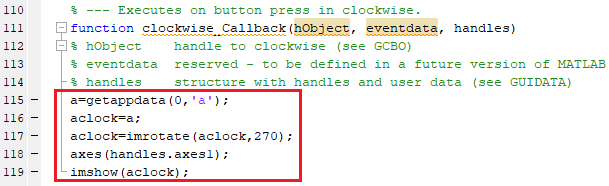8.      Rotate Image Anti-Clockwise

In the ‘anticlockwise’ function, copy and paste the below code to rotate the image in the anti-clockwise direction by using command imrotate(filename,‘angle’)

```a=getappdata(0,'a');
aclock=a;
aclock=imrotate(aclock,90);
axes(handles.axes1);
imshow(aclock);```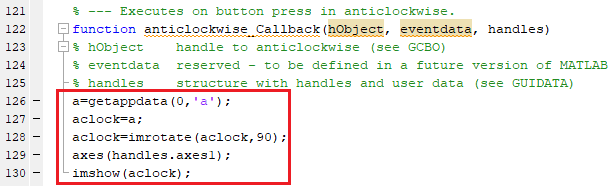### Run MATLAB GUI code for Image Processing

Now, click on the ‘RUN’ button to run the edited code in .m file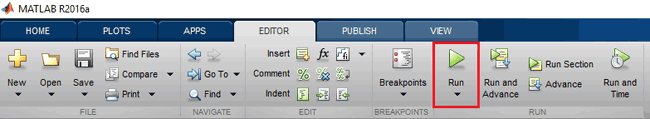MATLAB may take few seconds to respond, do not click on any GUI buttons until MATLAB is showing busy message in the lower left corner as shown below,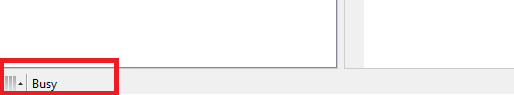When everything is ready, import the image from the PC by clicking on the ‘Upload Image’ button. Now, you will be able to convert or rotate the image by clicking any button accordingly. Below table will show you the task we are performing on the click of any particular button:The result on click of each button will give shown below,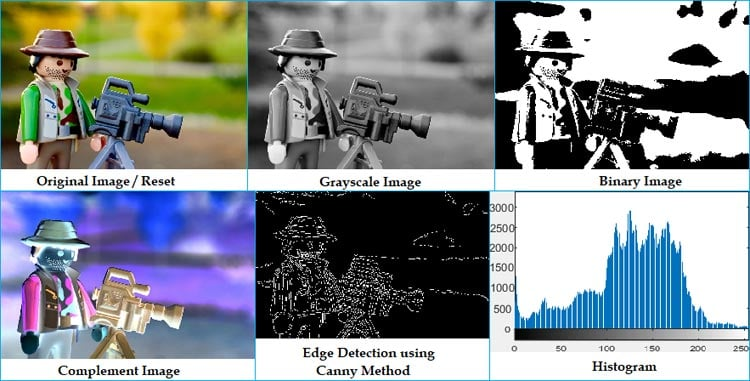Complete working of each button is demonstrated in the Video below.

You can even do advanced level of image processing with the Image Processing Toolbox which you can purchase from MATHWORKS official site, some of advance level operation are listed below:

• Geometric operations
• Block operations
• Linear filtering and filter design
• Transforms
• Image analysis and enhancement
• Binary image operations

### Nice Project. Can I please…

Nice Project. Can I please have the codes? Thank you.

Messan

### can I get source code please?

can I get source code please?